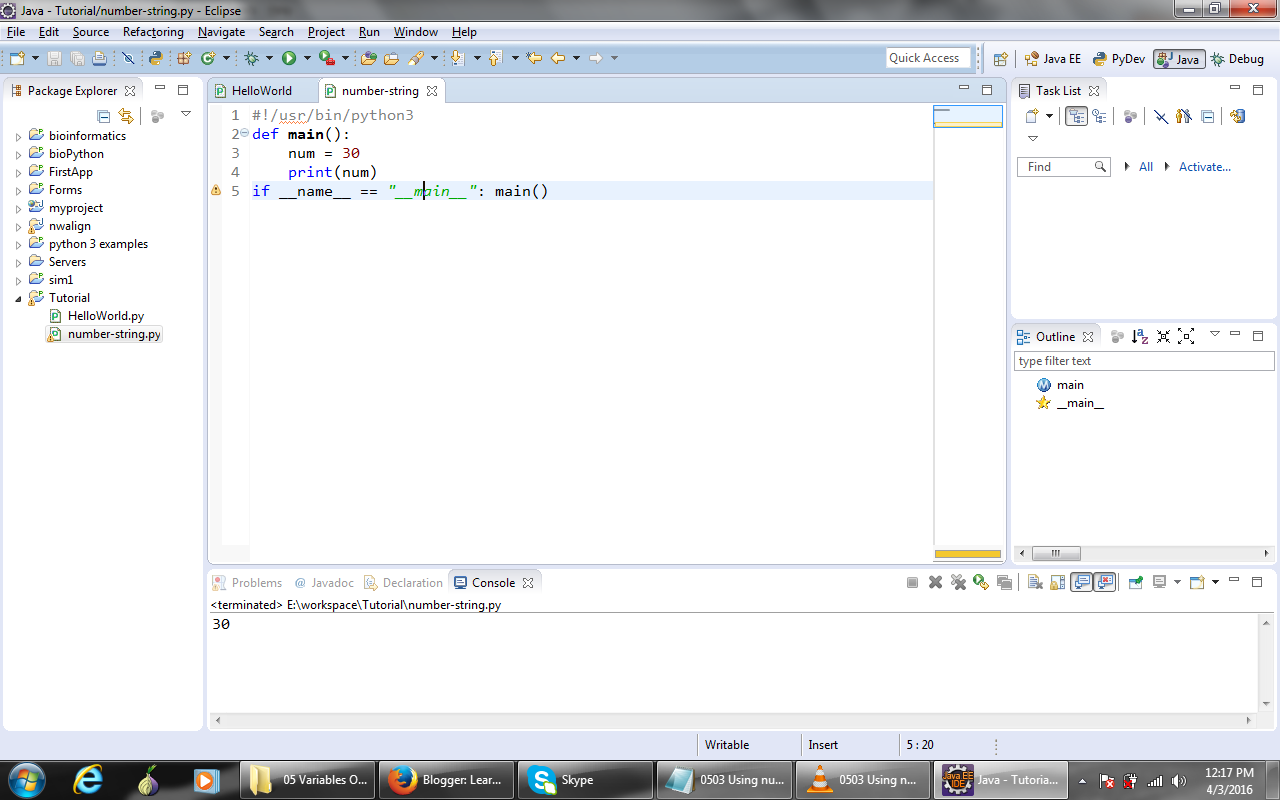# Numbers in python

Hello, how are you guys!!! I hope you will be fine and also enjoying this tutorial. In my previous post you learned about  python mutable and immutable objects in detail. You also learned the difference between mutable and immutable objects. Today you will learn "Numbers" in python with example.

Numbers in Python:
There are basically two different kinds of numbers in Python. Here in the below screen shot we define a variable. And we will  give it a value (num = 30). And then we will print that variable. You see we get that value.

1. #!/usr/bin/python3
2. def main():
3.    num = 30
4.    print(num)
5. if __name__ == "__main__": main()

Out Put:
30We can also check the type of number. In the code below in line no 4 we use "type()" function to check the type of a given number.
1. #!/usr/bin/python3
2. def main():
3.    num = 30
4.    print(type(num), num)
5. if __name__ == "__main__": main()

If we run the above code we will get both the type and the value (we see that its class is int, and its value is 30).

Out Put:
<class 'int'> 30

If we declare another variable like num1 and set its value to 30.0, now its type is going to be <class 'float' > 30.0. So those are the two different kinds of numbers in Python. In the below screen shot you see the difference between two.